# ISEE Middle Level Math : How to subtract variables

## Example Questions

1 2 3 5 Next →

### Example Question #143 : Operations

Subtract the following: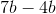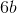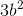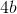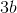Explanation:

To subtract variables, we will look at the variables like they are objects.  So, in the problemwe can think of the variable b as books.  So, we can write it like this: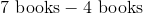We can read this as:  We have borrowed 7 books from the library.  We return 4 of those books to the library.  How many books do we have left?  We have 3 books.  So,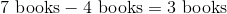We can subtract variables the same way.  We get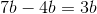### Example Question #144 : Operations

Simplify the following: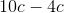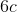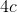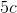Explanation:

When subtracting variables, we can look at the variables as objects.  So, in the problemwe can think of the variable as crackers.  So, we can write the problem like this: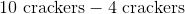Now, we can read the problem like this:  We have a plate with 10 crackers.  We go and eat 4 of the crackers.  How many crackers are left on the plate?  There are 6 crackers.  So,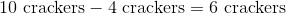We can subtract variables the same way: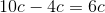### Example Question #145 : Operations

Subtract the following: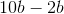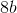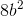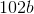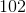Explanation:

When subtracting variables, we can look at the variables like objects.  So, in the problemwe can think of the variable b as brownies.  So, we can write the problem like this:We can read it like this:  You see there is a plate that contains 10 brownies.  You love brownies, so you eat 2 of them.  How many brownies are left on the plate?  There are 8 brownies left.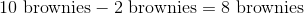We can subtract variables the same way.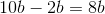### Example Question #146 : Operations

Subtract the following: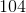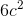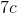Explanation:

To subtract variables, we will look at the variables like they are objects.  So, in the problemwe can think of the variable c as cookies.  So, we can write it like this: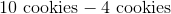We can read it like this:  There is a plate with 10 cookies on it.  They smell delicious, so we decide to eat 4 cookies.  How many cookies are left on the plate?  There are 6 cookies left on the plate.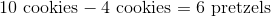We can subtract variables the same way:### Example Question #147 : Operations

Simplify: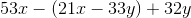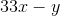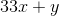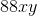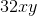Explanation:

Begin by distributing the subtraction through the group: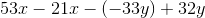Next, change the double negative to a positive: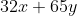1 2 3 5 Next →